Share

Books Shortlist

NCERT solutions for Class 9 Mathematics chapter 6 - Lines and Angles

Mathematics Textbook for Class 9

NCERT Mathematics Class 9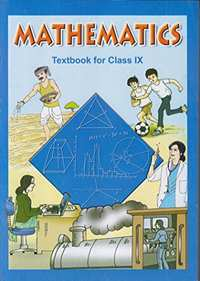Chapter 6: Lines and Angles

Ex. 6.10Ex. 6.20Ex. 6.30

Chapter 6: Lines and Angles Exercise 6.10 solutions [Pages 96 - 97]

Ex. 6.10 | Q 1 | Page 96

In the given figure, lines AB and CD intersect at O. If ∠AOC + ∠BOE = 70° and ∠BOD = 40°, find ∠BOE and reflex ∠COE.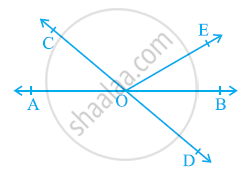Ex. 6.10 | Q 2 | Page 97

In the given figure, lines XY and MN intersect at O. If ∠POY = 90° and a:b = 2 : 3, find c.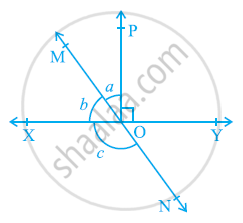Ex. 6.10 | Q 3 | Page 97

In the given figure, ∠PQR = ∠PRQ, then prove that ∠PQS = ∠PRT.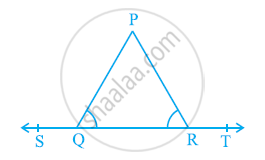Ex. 6.10 | Q 4 | Page 97

In the given figure, if x + y = w + z, then prove that AOB is a line.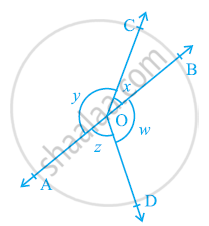Ex. 6.10 | Q 5 | Page 97

In the given figure, POQ is a line. Ray OR is perpendicular to line PQ. OS is another ray lying between rays OP and OR. Prove that ∠ROS = 1/2(∠QOS - ∠POS).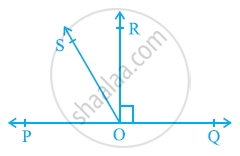Ex. 6.10 | Q 6 | Page 97

It is given that ∠XYZ = 64° and XY is produced to point P. Draw a figure from the given information. If ray YQ bisects ∠ZYP, find ∠XYQ and reflex ∠QYP.

Chapter 6: Lines and Angles Exercise 6.20 solutions [Pages 103 - 105]

Ex. 6.20 | Q 1 | Page 103

In the given figure, find the values of x and y and then show that AB || CD.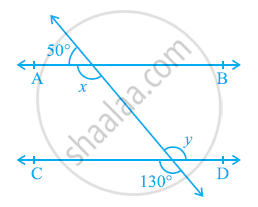Ex. 6.20 | Q 2 | Page 104

In the given figure, if AB || CD, CD || EF and y: z = 3: 7, find x.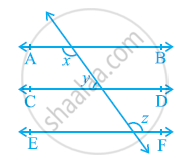Ex. 6.20 | Q 3 | Page 105

In the given figure, If AB || CD, EF ⊥ CD and ∠GED = 126º, find ∠AGE, ∠GEF and ∠FGE.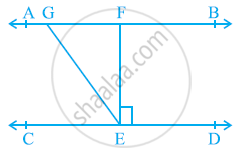Ex. 6.20 | Q 4 | Page 105

In the given figure, if PQ || ST, ∠PQR = 110º and ∠RST = 130º, find ∠QRS.

[Hint: Draw a line parallel to ST through point R.]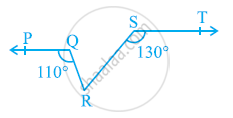Ex. 6.20 | Q 5 | Page 105

In the given figure, if AB || CD, ∠APQ = 50º and ∠PRD = 127º, find x and y.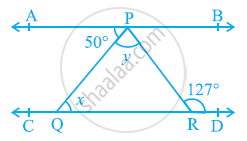Ex. 6.20 | Q 6 | Page 105

In the given figure, PQ and RS are two mirrors placed parallel to each other. An incident ray AB strikes the mirror PQ at B, the reflected ray moves along the path BC and strikes the mirror RS at C and again reflects back along CD. Prove that AB || CD.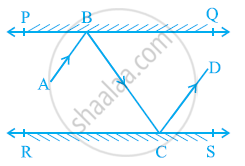Chapter 6: Lines and Angles Exercise 6.30 solutions [Pages 107 - 108]

Ex. 6.30 | Q 1 | Page 107

In the given figure, sides QP and RQ of ΔPQR are produced to points S and T respectively. If ∠SPR = 135º and ∠PQT = 110º, find ∠PRQ.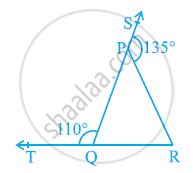Ex. 6.30 | Q 2 | Page 107

In the given figure, ∠X = 62º, ∠XYZ = 54º. If YO and ZO are the bisectors of ∠XYZ and ∠XZY respectively of ΔXYZ, find ∠OZY and ∠YOZ.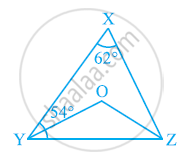Ex. 6.30 | Q 3 | Page 107

In the given figure, if AB || DE, ∠BAC = 35º and ∠CDE = 53º, find ∠DCE.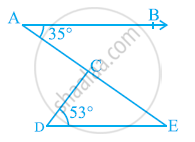Ex. 6.30 | Q 4 | Page 107

In the given figure, if lines PQ and RS intersect at point T, such that ∠PRT = 40º, ∠RPT = 95º and ∠TSQ = 75º, find ∠SQT.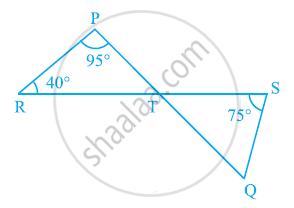Ex. 6.30 | Q 5 | Page 108

In the given figure, if PQ ⊥ PS, PQ || SR, ∠SQR = 28º and ∠QRT = 65º, then find the values of x and y.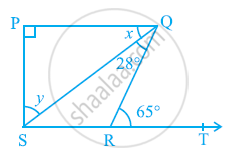Ex. 6.30 | Q 6 | Page 108

In the given figure, the side QR of ΔPQR is produced to a point S. If the bisectors of ∠PQR and ∠PRS meet at point T, then prove that ∠QTR = 1/2∠QPR.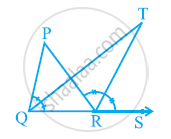Chapter 6: Lines and Angles

Ex. 6.10Ex. 6.20Ex. 6.30

NCERT Mathematics Class 9NCERT solutions for Class 9 Mathematics chapter 6 - Lines and Angles

NCERT solutions for Class 9 Maths chapter 6 (Lines and Angles) include all questions with solution and detail explanation. This will clear students doubts about any question and improve application skills while preparing for board exams. The detailed, step-by-step solutions will help you understand the concepts better and clear your confusions, if any. Shaalaa.com has the CBSE Mathematics Textbook for Class 9 solutions in a manner that help students grasp basic concepts better and faster.

Further, we at shaalaa.com are providing such solutions so that students can prepare for written exams. NCERT textbook solutions can be a core help for self-study and acts as a perfect self-help guidance for students.

Concepts covered in Class 9 Mathematics chapter 6 Lines and Angles are Angle Sum Property of a Triangle, Lines Parallel to the Same Line, Parallel Lines and a Transversal, Pairs of Angles, Intersecting Lines and Non-intersecting Lines, Basic Terms and Definitions, Concept to Lines and Angles.

Using NCERT Class 9 solutions Lines and Angles exercise by students are an easy way to prepare for the exams, as they involve solutions arranged chapter-wise also page wise. The questions involved in NCERT Solutions are important questions that can be asked in the final exam. Maximum students of CBSE Class 9 prefer NCERT Textbook Solutions to score more in exam.

Get the free view of chapter 6 Lines and Angles Class 9 extra questions for Maths and can use shaalaa.com to keep it handy for your exam preparation

S